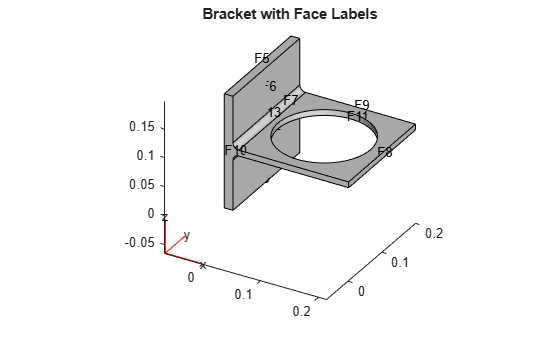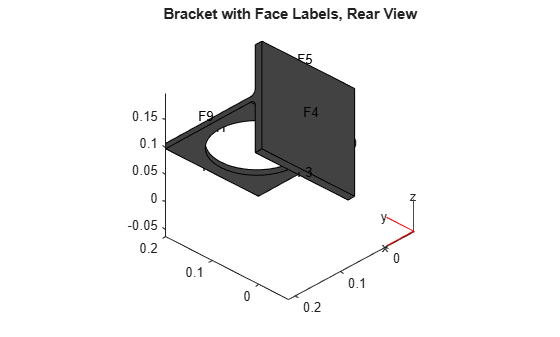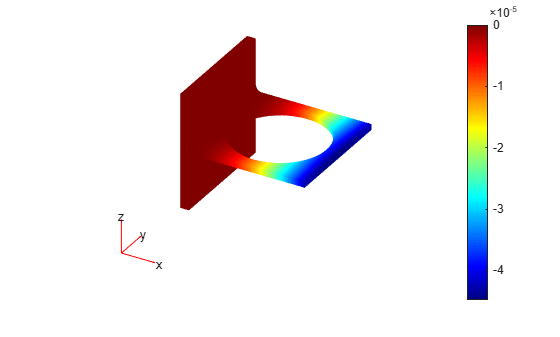StationaryResults

Time-independent PDE solution and derived quantities

Description

A StationaryResults object contains the solution of a PDE and its gradients in a form convenient for plotting and postprocessing.

• A StationaryResults object contains the solution and its gradient calculated at the nodes of the triangular or tetrahedral mesh, generated by generateMesh.

• Solution values at the nodes appear in the NodalSolution property.

• The array dimensions of NodalSolution, XGradients, YGradients, and ZGradients enable you to extract solution and gradient values for specified equation indices in a PDE system.

To interpolate the solution or its gradient to a custom grid (for example, specified by meshgrid), use interpolateSolution or evaluateGradient.

Creation

There are several ways to create a StationaryResults object:

• Solve a time-independent problem using the solvepde function. This function returns a PDE solution as a StationaryResults object. This is the recommended approach.

• Solve a time-independent problem using the assempde or pdenonlin function. Then use the createPDEResults function to obtain a StationaryResults object from a PDE solution returned by assempde or pdenonlin. Note that assempde and pdenonlin are legacy functions. They are not recommended for solving PDE problems.

Properties

expand all

Finite element mesh, returned as a FEMesh Properties object.

Solution values at the nodes, returned as a vector or array. For details about the dimensions of NodalSolution, see Dimensions of Solutions, Gradients, and Fluxes.

Data Types: double

x-component of the gradient at the nodes, returned as a vector or array. For details about the dimensions of XGradients, see Dimensions of Solutions, Gradients, and Fluxes.

Data Types: double

y-component of the gradient at the nodes, returned as a vector or array. For details about the dimensions of YGradients, see Dimensions of Solutions, Gradients, and Fluxes.

Data Types: double

z-component of the gradient at the nodes, returned as a vector or array. For details about the dimensions of ZGradients, see Dimensions of Solutions, Gradients, and Fluxes.

Data Types: double

Object Functions

 evaluateCGradient Evaluate flux of PDE solution evaluateGradient Evaluate gradients of PDE solutions at arbitrary points interpolateSolution Interpolate PDE solution to arbitrary points

Examples

collapse all

Create a PDE model for a system of three equations. Import the geometry of a bracket and plot the face labels.

model = createpde(3);
importGeometry(model,'BracketWithHole.stl');

figure
pdegplot(model,'FaceLabels','on')
view(30,30)
title('Bracket with Face Labels')figure
pdegplot(model,'FaceLabels','on')
view(-134,-32)
title('Bracket with Face Labels, Rear View')Set boundary conditions such that face 4 is immobile, and face 8 has a force in the negative z direction.

applyBoundaryCondition(model,'dirichlet','Face',4,'u',[0,0,0]);
applyBoundaryCondition(model,'neumann','Face',8,'g',[0,0,-1e4]);

Set coefficients that represent the equations of linear elasticity. See Linear Elasticity Equations.

E = 200e9;
nu = 0.3;
specifyCoefficients(model,'m',0,...
'd',0,...
'c',elasticityC3D(E,nu),...
'a',0,...
'f',[0;0;0]);

Create a mesh.

generateMesh(model,'Hmax',1e-2);

Solve the PDE.

results = solvepde(model)
results =
StationaryResults with properties:

NodalSolution: [14002x3 double]
Mesh: [1x1 FEMesh]

Access the solution at the nodal locations.

u = results.NodalSolution;

Plot the solution for the z-component, which is component 3.

pdeplot3D(model,'ColorMapData',u(:,3))Obtain a StationaryResults object from a legacy solver together with createPDEResults.

Create a PDE model for a system of three equations. Import the geometry of a bracket and plot the face labels.

model = createpde(3);
importGeometry(model,'BracketWithHole.stl');

figure
pdegplot(model,'FaceLabels','on')
view(30,30)
title('Bracket with Face Labels')figure
pdegplot(model,'FaceLabels','on')
view(-134,-32)
title('Bracket with Face Labels, Rear View')Set boundary conditions such that F4 is immobile, and F8 has a force in the negative z direction.

applyBoundaryCondition(model,'dirichlet','Face',4,'u',[0,0,0]);
applyBoundaryCondition(model,'neumann','Face',8,'g',[0,0,-1e4]);

Set coefficients for a legacy solver that represent the equations of linear elasticity. See Linear Elasticity Equations.

E = 200e9;
nu = 0.3;
c = elasticityC3D(E,nu);
a = 0;
f = [0;0;0];

Create a mesh.

generateMesh(model,'Hmax',1e-2);

Solve the problem using a legacy solver.

u = assempde(model,c,a,f);

Create a StationaryResults object from the solution.

results = createPDEResults(model,u)
results =
StationaryResults with properties:

NodalSolution: [14002x3 double]
Mesh: [1x1 FEMesh]

Access the solution at the nodal locations.

u = results.NodalSolution;

Plot the solution for the z-component, which is component 3.

pdeplot3D(model,'ColorMapData',u(:,3))Introduced in R2016a

Partial Differential Equation Toolbox DocumentationGet trial now